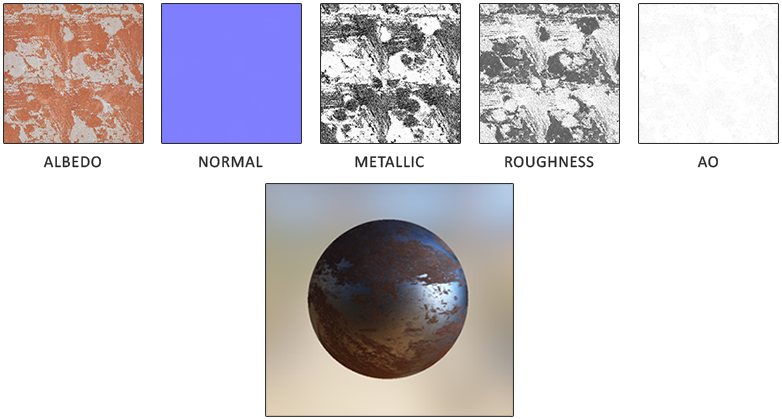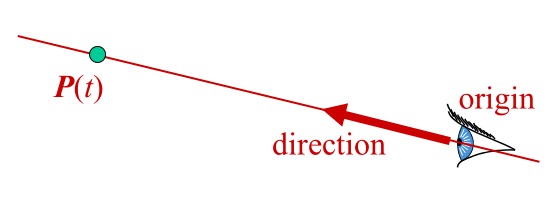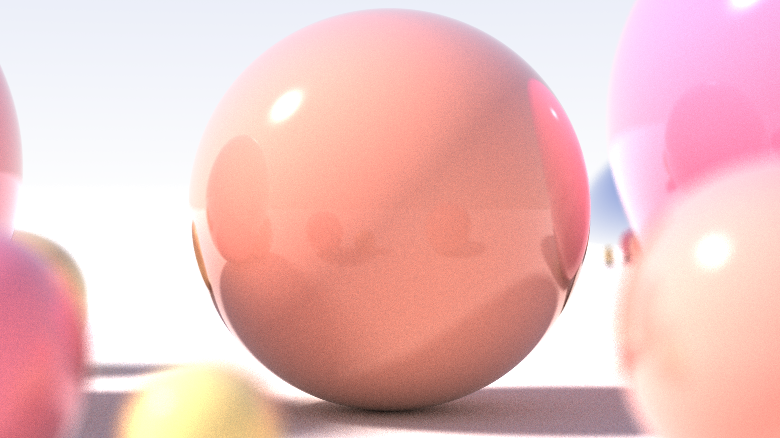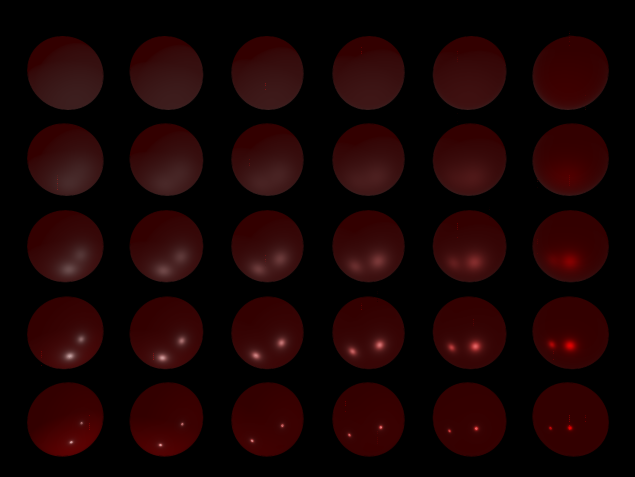# BRDF

The BRDF, or Bidirectional Reflective Distribution Function is a function that takes as input the incoming (light) direction $\omega_i$, the outgoing (view) direction $\omega_o$, the surface normal $n$ and a surface parameter a that represents the microsurface’s roughness. The BRDF approximates how much each individual light ray $\omega_i$ contributes to the final reflected light of an opaque surface given its material properties. For instance, if the surface has a perfectly smooth surface (like a mirror) the BRDF function would return 0.0 for all incoming light rays $\omega_i$ except the one ray that has the same (reflected) angle as the outgoing ray $\omega_o$ at which the function returns 1.0.

A BRDF approximates the material’s reflective and refractive properties based on the previously discussed microfacet theory. For a BRDF to be physically plausible it has to respect the law of energy conservation i.e. the sum of reflected light should never exceed the amount of incoming light. Technically, Blinn-Phong is considered a BRDF taking the same $\omega_i$ and $\omega_o$ as inputs. However, Blinn-Phong is not considered physically based as it doesn’t adhere to the energy conservation principle. There are several physically based BRDFs out there to approximate the surface’s reaction to light. However, almost all real-time render pipelines use a BRDF known as the Cook-Torrance BRDF.

The Cook-Torrance BRDF contains both a diffuse and specular part:

Share

# The Reflection Equation

## Overview

Physically based rendering strongly follows a more specialized version of the render equation known as the reflectance equation, which is the best model we have for simulating the visuals of light:

To understand the equation, we have to delve into a bit of radiometry. Radiometry is the measurement of electromagnetic radiation (including visible light). There are several radiometric quantities we can use to measure light over surfaces and directions, but we will only discuss a single one that’s relevant to the reflectance equation known as radiance, denoted here as $L$. Radiance is used to quantify the magnitude or strength of light coming from a single direction.

Share

# Theory of PBR## Overview

PBR (Physically Base Rendering) is a rendering technique that are more or less based on the same underlying theory which more closely matches that of the physical world. the biggest difference between the physical rendering model and the traditional rendering method is that it can more accurately describe and draw the interaction between the light and the surface of the object.

Share

# Do Ray Tracing## Generate Ray

Generate a ray that goes from the camera’s origin through the pixel location (pixelPosX, pixelPosY) of the camera. Note that pixelPosX and pixelPosY can be non-integer. The image origin is at the bottom-leftmost corner, that means:

• The bottom-leftmost corner of the image is (0, 0).
• The top-rightmost corner of the image is (imageWidth, imageHeight).
• The center of the bottom-leftmost pixel is (0.5, 0.5).
• The center of the top-rightmost pixel is (imageWidth-0.5, imageHeight-0.5).

Share

# Lighting and Materials

## Lighting

Caculate lighting effect is extremely complicated and it depends on way too many factors in real world, therefore we use a method based on approximations of reality using simplified models that are much easier to process and look relatively similar. These lighting models are based on the physics of light as we understand it. One of those models is called the Phong lighting model.

Phong lighting is a great and very efficient approximation of lighting, but its specular reflections break down in certain conditions, specifically when the shininess property is low resulting in a large (rough) specular area.Share

# Ray and Camera

## Ray

Finding ray-object intersection and computing surface normal is central to ray tracing.

Ray representations:

• Two 3D vectors
• Ray origin position
• Ray direction vector
• Parametric form
• $P(t) = origin + t \times direction$Share

# Various surfaces

## Ray-Plane Intersection

Plane is often represented in implicit form :

Equivalent:

where $N = [A B C]^T$ and $P = [x y z]^T$

To find ray-plane intersection, substitute ray equation $P(t)$ into plane equation:

1. We get $N \cdot P + D = 0$.
2. Sovle for $t$ to get $t_0$.
3. if $t_0$ is infinity, no intersection (ray is parallel to plane).
4. Intersection point is $P(t_0)$.
5. Verify that intersection is not behind ray origin.

The normal at the intersection is $N$ (or $-N$)

Share

# Ray Tracing Overview

## OverviewIn 3D compute graphics, ray tracing is rendering technique for generating an visual image by tracing the path of light. it has better effect than either ray casting and scan-line rendering techniques.

Typically, there are two question need be deal:

• What object has been seen?
• What color of the object is under the influence of light and environment?

In nature, a ray will travel to a surface that stop ray traveling, or until out of energy eventually disappears.
there are four things might happen with light ray: absorption, reflection, refraction, fluorescence,

Share

# Physically Based Rendering Catalogue

This is catalogue of Physically Based Rendering, I will update it if I have free time.(It’s means I don’t know what time I will finish one of them.)• Theory of Physically Based Rendering
• The Reflectance Equation
• BRDF
• Lighting
• IBL
Share

# Ray Tracing Catalogue

This is catalogue of Ray Tracing, I will update it if I have free time.(It’s means I don’t know what time I will finish one of them.)Coming soon…

• Ambient Occlusion
• Blur
Share